Mathematics and Computer Science
Volume 1, Issue 2, July 2016, Pages: 29-35

New Analytical Solutions for the Flow of a Fourth Grade Fluid Past a Porous Plate

Muhammet Yurusoy

Department of Mechanical Engineering, Afyon Kocatepe University, Afyon, Turkey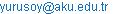Muhammet Yurusoy. New Analytical Solutions for the Flow of a Fourth Grade Fluid Past a Porous Plate. Mathematics and Computer Science. Vol. 1, No. 2, 2016, pp. 29-35. doi: 10.11648/j.mcs.20160102.12

Received: June 28, 2016; Accepted: August 5, 2016; Published: August 25, 2016

Abstract: The flow of fourth grade fluid flow over a porous plate with heat transfer is considered. By using the perturbation techniques, approximate analytical solutions for velocity and temperature profiles have been obtained. Comparing with the Newtonian effect, it turns out that if the second grade, third grade and fourth grade effects are small, an ordinary perturbation problem occurs. To find fourth grade fluids, velocity and temperature profiles, which are attained, are compared with numerical solutions. The approximate solutions run in well with the numerical solutions. This is to demonstrate us that the perturbation technique is a robust tool to find great approximations to nonlinear equations of fourth grade fluids. Velocity and temperature profiles are calculated for diverse second grade, third grade and fourth grade non-Newtonian fluid parameters.

Keywords: Fourth Grade Fluid Equations, Boundary Layer Analysis, Perturbation Methods

Contents

1. Introduction

There is a significant industrial application for the heat transfer from the surface such as molten plastics, polymers or slurries. Theoretical and experimental studies are used to have a better understanding of these applications. Mathematical models of these applications classified by considerably high non-linear ordinary or partial differential equations. There are so many studies as to the Newtonian fluids. However, even though non-Newtonian fluids have heavily used in the industry and technology, they are not sufficiently placed important. Having been developed to solve the non-linear equations, Non-Newtonian fluid mechanics pose a great opportunity to work on the mathematical techniques. There are a great deal of models to explain the non-Newtonian behavior of the fluids. The power-law, differential-type and rate-type models are the ones which are gained much recognition. Boundary layer assumptions were successfully applied to these models and a lot of work has been done on them. Rivlin–Ericksen type of fluids which is a non- Newtonian class has gained recognition and is used to solve a number of flow problems. So-called ‘Third-Grade Fluids’ or "Fourth-Grade Fluids" is a particular type of this class. To explain the non-Newtonian behavior in fluids, fourth grade fluids are not commonly used.

Rajagopal and Fosdick has developed the theory of third-grade fluids . Rajagopal  studied on the stability characteristics of such fluids. Szeri and Rajagopal  observed their flow between heated parallel plates. Rajagopal and Szeri  worked on a third grade fluid past a porous plate. From ordinary perturbation solutions to arbitrary orders of approximation alongside with the numerical solutions were submitted for the problem. Furthermore, by considering heat transfer, Maneschy et al.  improved the numerical solutions . A similar study work has been done . Using homotopy analysis method, they were able to solve the porous plate problem and brought forward analytical solutions for the generalized second grade fluids. It is not until now, Lie Group analysis for the boundary layer equations of the modified second grade fluid has been submitted . Using perturbation method, Pakdemirli et al.  figured out the porous plate problem and submitted an analytical and numerical solutions of modified second grade fluid model. To solve the differential equation, perturbation method is mainly used. Massoudi and Christie  observed the pipe flow of third-grade fluids and figured out the momentum and energy equations on a numerical basis. Yürüsoy and Pakdemirli  examined the third-grade fluid flow in pipes again. They presented a perturbation method and solved the flow equations in an analytic manner. To study third-grade fluid flow over a porous wall, Hayat et al.  incorporated Lie Group theory with perturbations.

By using the perturbation method, approximate analytical solutions of fourth grade fluids flow a past porous plate with heat transfer are submitted and they are compared with the numerical solutions in this study. It is presumed that the surface temperature is higher than fluid temperature. By keeping the constant viscosity, Fourth-grade fluid has been applied to explain the non-Newtonian effect. Motion equations in dimensionless form are originated. Comparing with the Newtonian effect, it turns out that if the second grade, third grade and fourth grade effects are small, an ordinary perturbation problem occurs. For the solution, the validity criterion is determined. For various non-Newtonian parameters, velocity and temperature profiles are calculated.

2. Equations of Motions

Mass conservation, linear momentum equation and energy equations are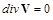(1)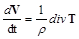(2)(3)

where r is the fluid density, k is thermal conductivity, cp is the specific heat, V is the velocity vector, q is the temperature and T is the Cauchy stress tensor. We have for the fourth grade fluid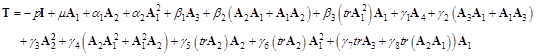(4)

where p is pressure, I is the identity matrix, m is the viscosity, α1, α2, β1, β2, β3, γ1, γ2, γ3, γ4, γ5, γ6, γ7 and γ8 are the material constant. A1, A2, A3 and A4 are Rivlin-Ericksen tensors. The tensors are defined as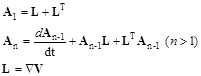(5)

For the model, when αi=0, βi=0 and γi=0, the fluid is Newtonian. βi=0 and γi=0 equivalent to the second grade fluid, γi=0 equivalent to the third grade fluid.

For the particular case of flow over a porous plate, it is presumed that the velocity components are dependent only on the y coordinate i.e. u = u(y) and v = v(y). Considering this assumption, from the continuity equation (1)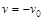(6)

where v0 is a constant which represents the suction (v0 > 0) or injection velocity (v0 < 0). Inserting the assumed profiles to the momentum and the energy equations yields after simplifications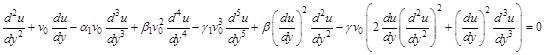(7)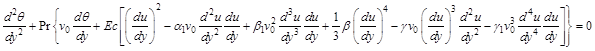(8)

where the emerging dimensionless variables and parameters are defined by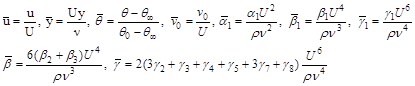(9)

in which U is a reference velocity, θ0 is the wall temperature, θ is the temperature of the free stream and ν=μ/ρ is the fluid kinematic viscosity. Pr=μcp/k, Ec=U2/cp(θ0-θ) are respectively the Prandtl and Eckert numbers and bars have been suppressed throughout. We will solve equations (7) and (8) in the domain y[0,) with the following boundary conditions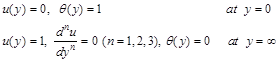(10)

In following section, by using the perturbation theory velocity and temperature profiles are figured out.

3. Perturbation Solutions

For the system (7) and (8) with boundary condition (10), perturbation solutions will be studied. Under the weakly non-linear assumption, the non-linear terms as well as the non-homogenous terms of equation (7) are chosen to be small when compared to other terms, that is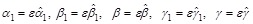(11)

where ε is the perturbation parameter (ε <<1). The approximate velocity and temperature profiles can be written as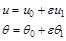(12)

Substituting equations (11) and (12) into equations (7), (8) and (10) and separating at each order of ε, one has

Order 1: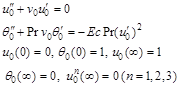(13)

Order ε: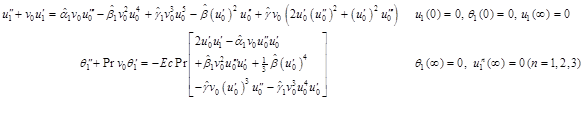(14)

For the first order, the solutions are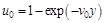(15)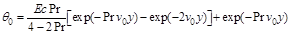(16)

Substituting these solutions into order ε equations, one finally obtains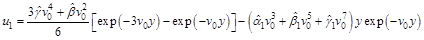(17)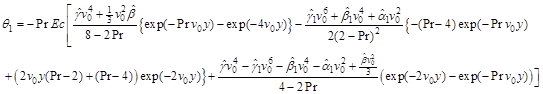(18)

Substituting (15)-(18) into the expansions given in (12), returning back to the original parameters since ε is artificially introduced, one has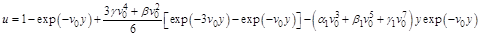(19)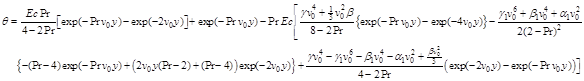(20)

Perturbation solutions (19) and (20) will be compared with numerical solution in the following section.

4. Numerical Results and Comparisons

In this section, analytical solutions figures will be demonstrated. The perturbation solutions will be compared with the numerical solutions of the equations. Matlab package bvp4c using of finite difference techniques, is applied in numerical solutions.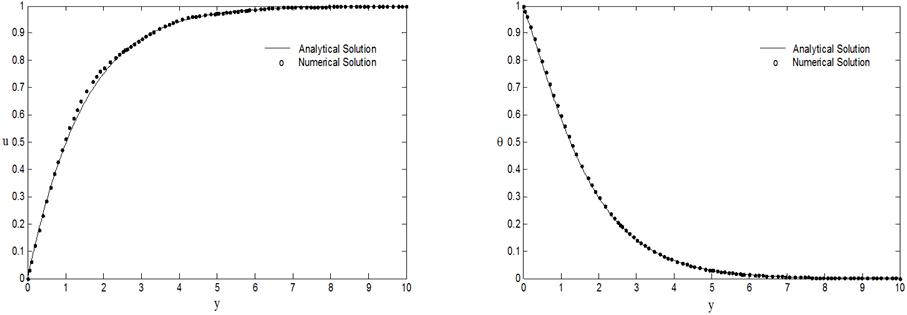Figure 1. Comparison of perturbation solutions with numerical solutions for the velocity and temperature variations. (v0=1, Pr=1, Ec=1; γ=0.1, γ1=0.1, α1=0.1, β=0.1, β1=0.1).Figure 2. Effect of suction parameter v0 on the velocity and temperature profiles. (Pr=1, Ec=1; γ=0.1, γ1=0.1, α1=0.1, β=0.1, β1=0.1).Figure 3. Effect of material parameter a1 on the velocity and temperature profiles. (v0=1, Pr=3, Ec=1; γ=0.1, γ1=0.1, β=0.1, β1=0.1).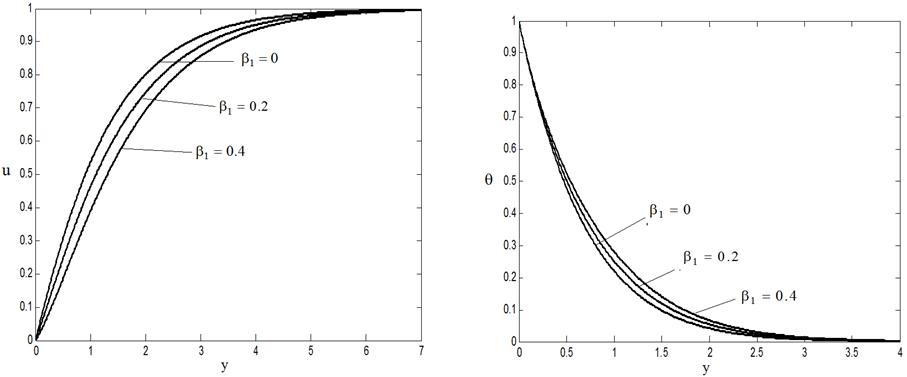Figure 4. Effect of material parameter b1 on the velocity and temperature profiles. (v0=1, Pr=3, Ec=1; γ=0.1, γ1=0.1, β=0.1, α1=0.1).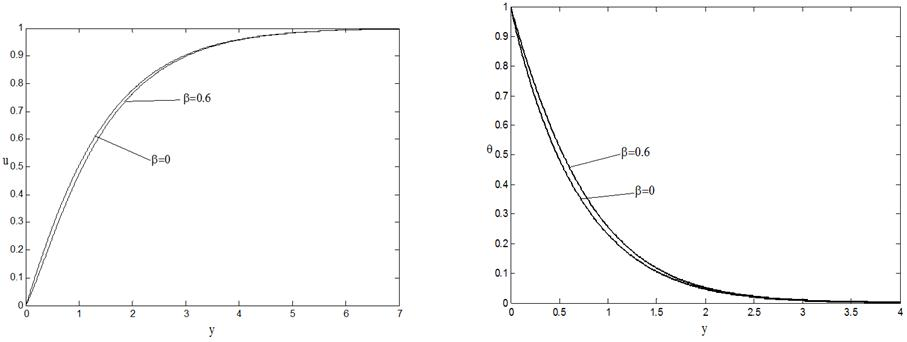Figure 5. Effect of material parameter b on the velocity and temperature profiles. (v0=1, Pr=3, Ec=1; γ=0.1, γ1=0.1, β1=0.1, α1=0.1).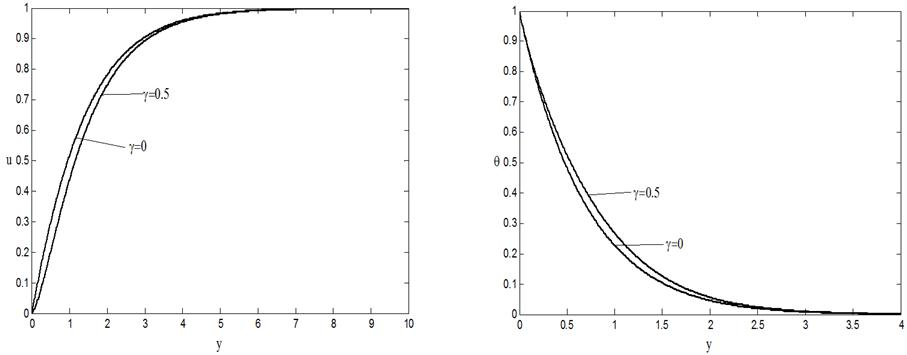Figure 6. Effect of material parameter g on the velocity and temperature profiles. (v0=1, Pr=3, Ec=1; β=0.1, γ1=0.1, β1=0.1, α1=0.1).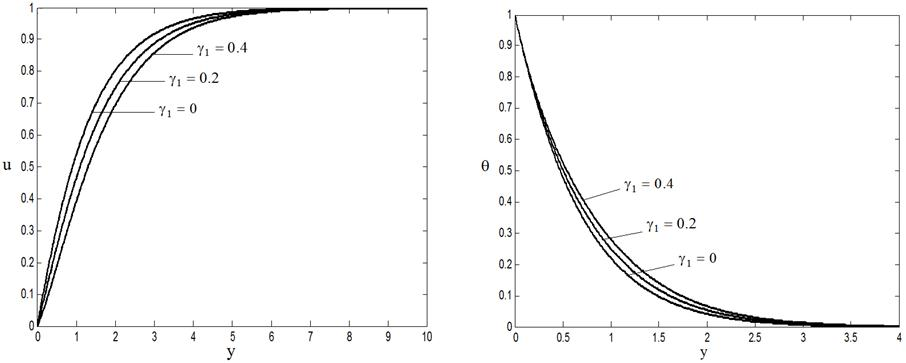Figure 7. Effect of material parameter g1 on the velocity and temperature profiles. (v0=1, Pr=3, Ec=1; β=0.1, γ=0.1, β1=0.1, α1=0.1).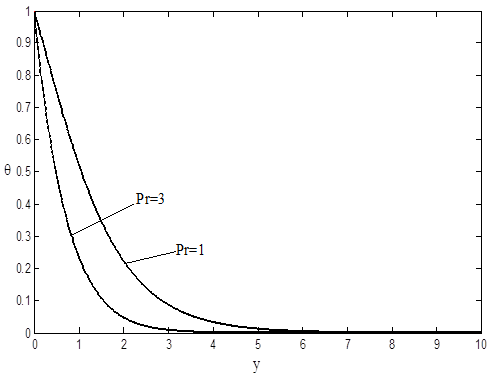Figure 8. Effect of Prandtl number on the temperature profile. (v0=1, Ec=1; β=0.1, γ=0.1, γ1=0.1, β1=0.1, α1=0.1).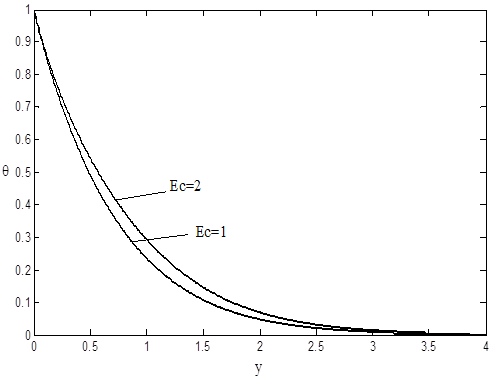Figure 9. Effect of Eckert number on the temperature profile. (v0=1, Pr=3; β=0.1, γ=0.1, γ1=0.1, β1=0.1, α1=0.1).

Figure 1 shows the comparison between the Analytical and numerical solutions. The harmony there is very well and points equivalent to numerical solutions are demonstrated as circles to make a distinction between them. As you can see there is a separation between the solutions for high values of the material parameters, due to the fact that it is presumed that the value of material parameters are very low for perturbation solutions. Figure 2 shows the effects of a suction parameter on the velocity and temperature profiles. The more suction parameter increases, the more velocity increases. What’s more, the boundary layer thickness for the temperature profiles decreases once suction parameter is increased. Furthermore, suction parameter gives way to a decrease in thermal and velocity boundary layer thickness, as well. Therefore, it is concluded that to control the boundary layer thickness, the suction on the plate can be useful. As a matter of fact, there is relative interest in flows with suction or injection for boundary layer control. It is clear when we observe in physical fashion that suction and injection give way to opposite effects on the boundary layer flows. As we have already observed that the suction causes thickening of the boundary layer, when the suction velocity is robust in a sufficient manner. For that reason, boundary layer thickness decreases if we increase the suction velocity. Figure (3-7) shows the effects of materials parameters (γ, γ1, α1, β, β1) on the velocity and temperature profiles. The effects of materials parameters, both the temperature and velocity profiles, have opposite effect on that suction velocity, for example, the more velocity and thermal boundary layer thickness increase the more materials parameters are increased. Figure 8 shows the effects of Prandtl number on the temperature profiles. When an increase occurs in Prandtl number, it decreases temperatures. Figure 9 depicts the effects of Eckert number on the temperature. If a decrease occurs in Eckert number, it increases temperatures.

5. Conclusion Remarks

In this study, a comprehensive perturbation analysis for the flow and heat transfer of a fourth grade fluid over a porous plate has been demonstrated. By using perturbation method, the considerably high non-linear ordinary differential equations are figured out in an analytical manner. When compared to other terms, in parallel with the weak non-linear assumption, the non-linear terms alongside with the non-homogenous terms of equations of motion are chosen to be small. The effects of non-Newtonian materials respective parameters on the velocity and temperature profiles have been discussed. When we examine expressed solutions, they are run well with the numerical ones in the scope of the validity of the analytic solutions. The effects of materials parameters, both the temperature and velocity profiles, have opposite effect on that suction velocity, for example, the more velocity and thermal boundary layer thickness increase the more materials parameters are increased. In conclusion, When an increase occurs in Prandtl number, it decreases temperatures, but for Eckert number, a reverse effect has been observed.

References

1. R. L. Fosdick and K. R. Rajagopal, Thermodynamics and stability of fluids of third grade, Proceedings of the Royal Society A 1, 351-377, 1980.
2. K. R. Rajagopal, On the stability of third-grade fluids, Arch. Mech. 32, 867-875. 1980.
3. A. Z. Szeri and K. R. Rajagopal, Flow of a non-Newtonian fluid between heated parallel plates, Int. J. Non-Linear Mech. 20, 91-101, 1985.
4. K. R. Rajagopal, A. Z. Szeri and W. Troy, An existence theorem for the flow of a non-Newtonian fluid past an infinite porous plate, Int. J. Non-Linear Mech. 21, 279-289. 1986.
5. C. E. Maneschy, M. Massoudi and A. Ghoneimy, Heat transfer analysis of a non-Newtonian fluid past a porous plate, International Journal of Non-Linear Mechanics, 28, 131-143, 1993.
6. T. Hayat and M. Khan, Homotopy solutions for a generalized second-grade fluid past a porous plate, Nonlinear Dynamics, 42, 395-405, 2005.
7. M. Pakdemirli, T. Hayat, M. Yürüsoy, S. Abbasbandy and S. Asghar, Perturbation analysis of a modified second grade fluid over a porous plate ,Nonlinear Analysis: Real World Applications, 12, 1774-1785, 2011.
8. M. Massoudi and I. Christie, Effects of variable viscosity and viscous dissipation on the flow of a third grade fluid in a pipe, Int. J. Non-Linear Mech. 30, 687-699, 1995.
9. M. Yurusoy and M. Pakdemirli, Approximate analytical solutions for the flow of a third-grade fluid in a pipe. Int. J. Nonlinear Mech. 37, 187–195, 2002.
10. T. Hayat, A. H. Kara, and E. Momoniat, Exact flow of a third grade fluid on a porous wall. Int. J.Non-LinearMech.38, 1533–1537, 2003.

 Contents 1. 2. 3. 4. 5.
Article ToolsAbstractPDF(2421K)